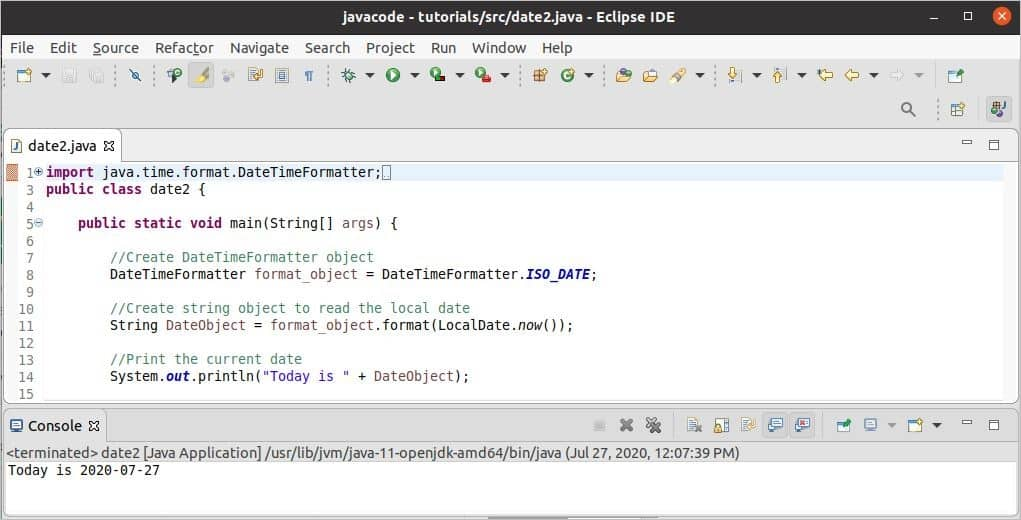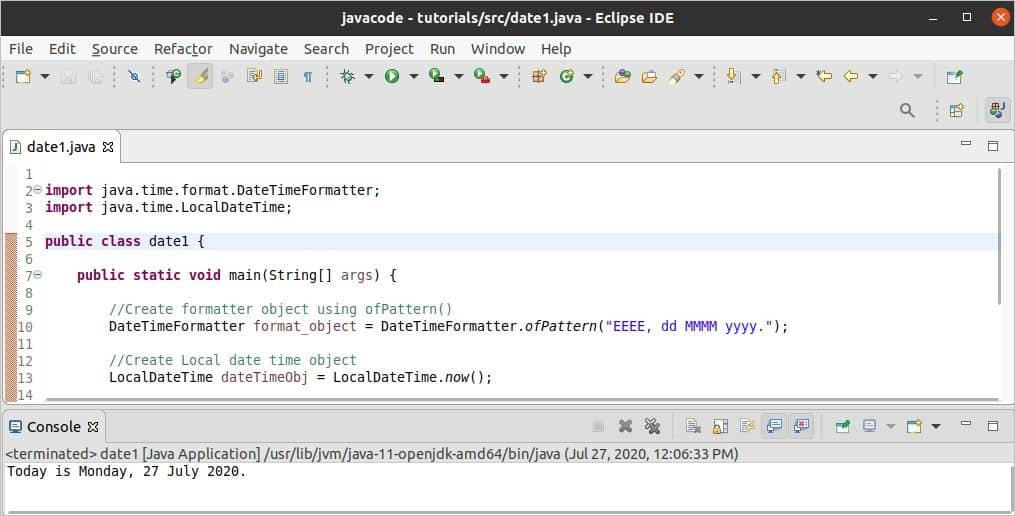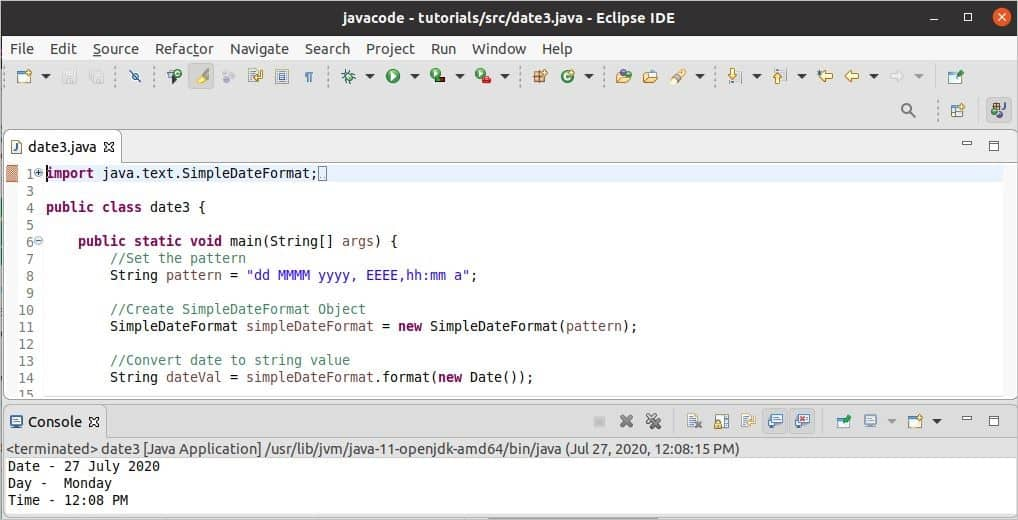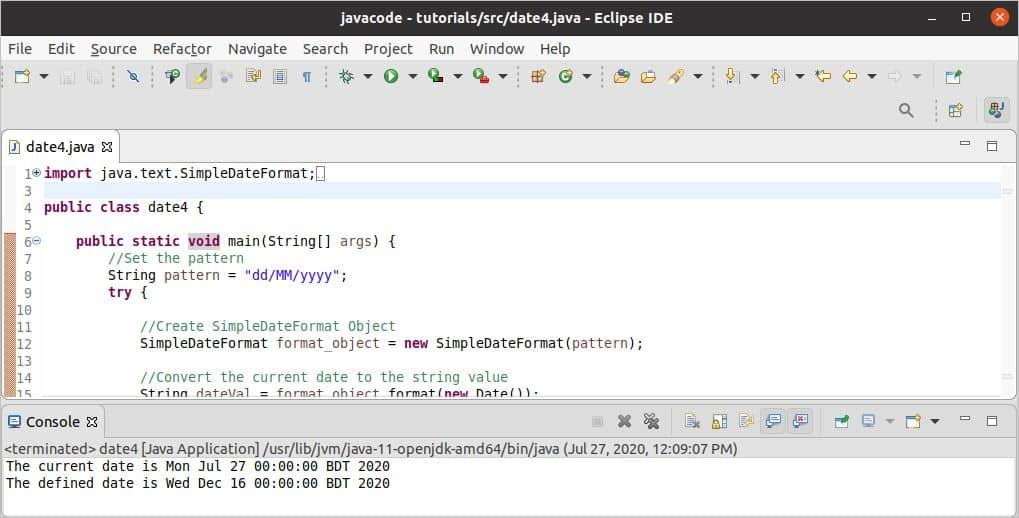Java

# Java date format

Date value needs to format for various programming purposes. One of the essential reasons to format the date is to represent the date value in a human-readable format. Many classes exist in Java to format the date value. DateTimeFormatter and SimpleDateFormat are two of them. These classes can be used to format date values in different ways. How these two classes can be used to format the date in Java is explained in this tutorial.

### DateTimeFormatter:

It is introduced in Java 8 to format date value. It is used to format ZoneDateTime, LocalDateTime, LocalDate, and LocalTime. The DateTimeFormatter object can be created by using an inbuilt pattern, custom pattern, and localized style. Some uses of this class are shown in this tutorial with multiple examples.

### SimpleDateFormat:

It is used to format and parse date values with local support. The date to string and string to date conversion can be done easily by using this class. It is created by extending the DateFormat class. Some uses of this class are shown in this tutorial with multiple examples.

### Example-1: Format date using DateTimeFormatter and inbuilt pattern

The date and time format of DateTimeFormatter is fixed for the inbuilt pattern. The date and time value will be printed based on the inbuilt pattern used in the code. ISO_DATE pattern is used in the following code that prints the date in the format, yyyy-mm-dd. The current date is read here by using the method named now() of the LocalDate class that returns the formatted date value in the string.

import java.time.format.DateTimeFormatter;
import java.time.LocalDate;
public class date2 {

public static void main(String[] args) {

//Create DateTimeFormatter object
DateTimeFormatter format_object = DateTimeFormatter.ISO_DATE;

//Create string object to read the local date
String DateObject = format_object.format(LocalDate.now());

//Print the current date
System.out.println("Today is " + DateObject);
}
}

Output:

The following image shows the output of the above code. The output will depend on the current system date.### Example-2: Format date using DateTimeFormatter and custom pattern

You can print the date value in a more human-readable format by using the custom pattern of DateTimeFormatter. The pattern used in the following example will print the current weekday with the date value. ofPattern() method is used here to set the pattern of the date value. In the pattern, ‘EEEE’ is used to print the weekday in full form, ‘dd’ is used to print the day, ‘MMMM‘ is used to print the month’s name in full form and ‘yyyy’ is used to print the four digits year. The now() method of LocalDateTime is used here to read the current system date and time. The format() method is used to return the formatted date value in string based on the pattern.

import java.time.format.DateTimeFormatter;
import java.time.LocalDateTime;

public class date1 {

public static void main(String[] args) {

//Create formatter object using ofPattern()
DateTimeFormatter format_object = DateTimeFormatter.ofPattern("EEEE, dd MMMM yyyy.");

//Create a Local date-time object
LocalDateTime dateTimeObj = LocalDateTime.now();

//Get formatted String
String stringDate = format_object.format(dateTimeObj);

//Print the formatted date
System.out.println("Today is " + stringDate);
}
}

Output:

The following image shows the output of the above code. The output will depend on the current system date.### Example-3: Format date using SimpleTimeFormat and format() method

The following example shows the use of SimpleTimeFormat to print the formatted date and time value based on the used pattern. Here, “dd MMMM yyyy, EEEE, hh:mm a” is used as a pattern in the code. The meaning of each part of the pattern without  ‘hh’ and ‘mm’ is explained in the previous example. ‘hh’ is used here to print the current hour value, and ‘mm’ is used to print the current minute value. Date class is used in the code to read the current system date and time. The format() method is used to return the formatted date and time values in string based on the used pattern.

import java.text.SimpleDateFormat;
import java.util.Date;

public class date3 {
public static void main(String[] args) {
//Set the pattern
String pattern = "dd MMMM yyyy, EEEE,hh:mm a";

//Create SimpleDateFormat Object
SimpleDateFormat simpleDateFormat = new SimpleDateFormat(pattern);

//Convert date to the string value
String dateVal = simpleDateFormat.format(new Date());

//Split the date value based on comma(,)
String[] today_arr = dateVal.split(",");

//Print the formatted output
System.out.println("Date - " + today_arr+ "\nDay - " + today_arr +
"\nTime - " + today_arr);
}
}

Output:

The following image shows the output of the above code. The output will depend on the current system date and time.### Example-4: Format date using SimpleTimeFormat and  parse() method

The following example shows the use of the parse() method to read the current date or a  particular date that converts the formatted string value into a date value. Here, the “dd/MM/yyyy” pattern is used to set the particular date for the parse() method. The current date value is read by Date class and returned the value as a string. This value is again converted into date value by using the parse() method. Next, a particular date value is set as a string in the parse() method according to the pattern. The value is converted into a date like before.

import java.text.SimpleDateFormat;
import java.util.Date;

public class date4 {

public static void main(String[] args) {
//Set the pattern
String pattern = "dd/MM/yyyy";
try {

//Create SimpleDateFormat Object
SimpleDateFormat format_object = new SimpleDateFormat(pattern);

//Convert the current date to the string value
String dateVal = format_object.format(new Date());

//Convert the string to date value
Date date1 = format_object.parse(dateVal);

//Print the date value
System.out.println("The current date is "+ date1);

//Convert the defined date string to date value
Date date2 = format_object.parse("16/12/2020");

//Print the date value
System.out.println("The defined date is " + date2);
}
catch (java.text.ParseException e) {
//Print error message
System.err.println(e.getMessage());
}
}
}

Output:

The following output will be appeared after running the script, and the first output will depend on the current system time.### Conclusion:

Different ways of formatting date value are shown in this tutorial by using various Java classes. The tutorial mainly focuses on the formatting of the date values. You can format the time value also by the classes used here. I hope, date formatting task in Java will be easier after reading this tutorial.